Select Page

# 12 Science CBSE Solutions for MCQ Maths Continuity and Differentiability in English

12 Science CBSE Solutions for MCQ Maths Continuity and Differentiability in English to enable students to get Solutions in a narrative video format for the specific question.

Expert Teacher provides 12 Science CBSE Solutions for MCQ Maths Continuity and Differentiability through Video Solutions in English language. This video solution will be useful for students to understand how to write an answer in exam in order to score more marks. This teacher uses a narrative style for a question from Continuity and Differentiability not only to explain the proper method of answering question, but deriving right answer too.

Please find the question below and view the Solution in a narrative video format.

Question:

Solution Video in English:

You can select video Solutions from other languages also. Please check Solutions in ( Hindi )

## Similar Questions from CBSE, 12th Science, Maths, Continuity and Differentiability

Question 1 : Findfor the function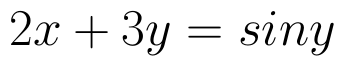. (View Answer Video)

Question 2 : Differentiate w.r.t.x the function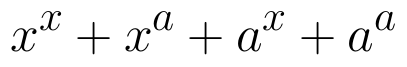, for some fixed a > 0 and x > 0. (View Answer Video)

Question 3 :  Find the second order derivative of the function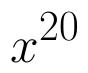. (View Answer Video)

Question 4 : If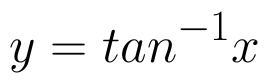, find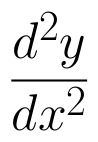in terms of y alone. (View Answer Video)

Question 5 : Differentiate w.r.t.x the function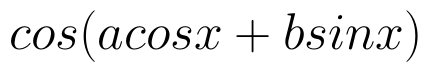, for some constant a and b. (View Answer Video)

### Determinant

Question 1 :  Find the area of the triangle with vertices at the points (-2, -3), (3, 2), (-1, -8). (View Answer Video)

Question 2 :  Evaluate the determinants: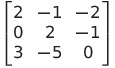. (View Answer Video)

Question 3 : A system of linear equations AX = B is said to be inconsistent, if the system of equations has, (View Answer Video)

Question 4 : If A is an invertible matrix of order 2, then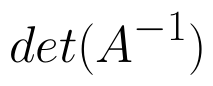is equal to, (View Answer Video)

Question 5 : If x, y, z are non-zero real numbers, then the inverse of matrix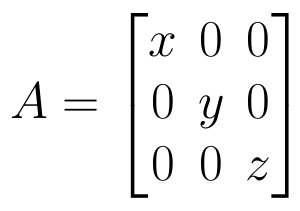is, (View Answer Video)

### Vector Algebra

Question 1 : Find a vector in the direction of vector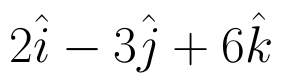which has magnitude 21 units.  (View Answer Video)

Question 2 : Find the vector quantities from the following:
(i) 10 kg          (ii) 2 m north-west          (iii)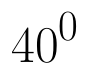(iv) 40 W         (v)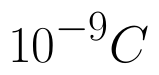(vi)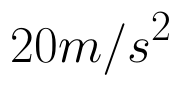(View Answer Video)

Question 3 : For given vectors,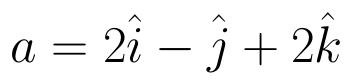and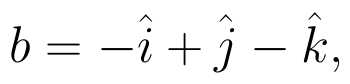find the unit vector in the direction of the vector a + b. (View Answer Video)

Question 4 :  Find the sum of the vectors :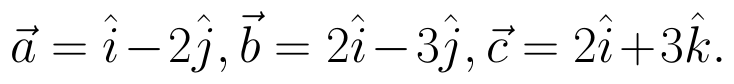(View Answer Video)

Question 5 : Find the sum of the vectors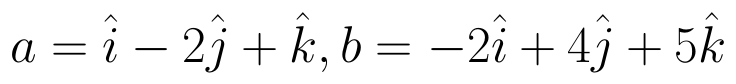and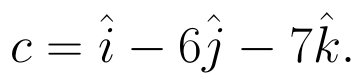(View Answer Video)

### Three Dimensional Geometry

Question 1 : Find the equation of the plane passing through the line of intersection of the planes x + y + z = 1 and 2x + 3y + 4z = 5 which is perpendicular to the plane x - y + z = 0. Also, find the distance of the plane obtained above from the origin. (View Answer Video)

Question 2 : If a line has the direction ratios -18, 12, -4, then what are its direction cosines? (View Answer Video)

Question 3 : Find the distance of the point (1, -2, 3) from the plane x-y+z=5 measured parallel to the line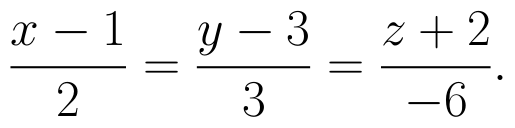(View Answer Video)

Question 4 : Find the equation of the plane with intercept 3 on the y-axis and parallel to ZOX plane. (View Answer Video)

Question 5 : Write the vector equation of the plane passing through the point (a, b, c) and parallel to the plane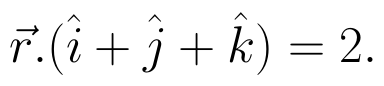(View Answer Video)